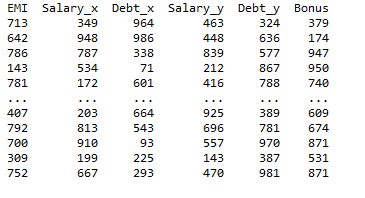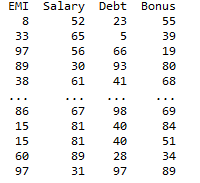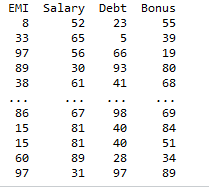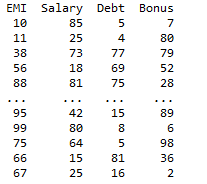Open in App
Not now

# Prevent duplicated columns when joining two Pandas DataFrames

• Difficulty Level : Hard
• Last Updated : 18 Nov, 2022

Column duplication usually occurs when the two data frames have columns with the same name and when the columns are not used in the JOIN statement. In this article, let us discuss the three different methods in which we can prevent duplication of columns when joining two data frames.

Syntax:

`pandas.merge(left, right, how='inner', on=None, left_on=None, right_on=None)`

Explanation:

• left – Dataframe which has to be joined from left
• right – Dataframe which has to be joined from the right
• how – specifies the type of join. left, right, outer, inner, cross
• on – Column names to join the two dataframes.
• left_on – Column names to join on in the left DataFrame.
• right_on – Column names to join on in the right DataFrame.

## Normally merge:

When we join a dataset using pd.merge() function with type ‘inner’, the output will have prefix and suffix attached to the identical columns on two data frames, as shown in the output.

## Python3

 `# import python pandas package` `import` `pandas as pd`   `# import the numpy package` `import` `numpy as np`   `# Create sample dataframe data1 and data2` `data1 ``=` `pd.DataFrame(np.random.randint(``1000``, size``=``(``1000``, ``3``)),` `                     ``columns``=``[``'EMI'``, ``'Salary'``, ``'Debt'``])` `data2 ``=` `pd.DataFrame(np.random.randint(``1000``, size``=``(``1000``, ``3``)),` `                     ``columns``=``[``'Salary'``, ``'Debt'``, ``'Bonus'``])`   `# Merge the DataFrames` `merged ``=` `pd.merge(data1, data2, how``=``'inner'``, left_index``=``True``,` `                  ``right_index``=``True``)` `print``(merged)`

Output:## Method 1: Use the columns that have the same names in the join statement

In this approach to prevent duplicated columns from joining the two data frames, the user needs simply needs to use the pd.merge() function and pass its parameters as they join it using the inner join and the column names that are to be joined on from left and right data frames in python.

Example:

In this example, we first create a sample dataframe data1 and data2 using the pd.DataFrame function as shown and then using the pd.merge() function to join the two data frames by inner join and explicitly mention the column names that are to be joined on from left and right data frames.

## Python3

 `# import python pandas package` `import` `pandas as pd`   `# import the numpy package` `import` `numpy as np`   `# Create sample dataframe data1 and data2` `data1 ``=` `pd.DataFrame(np.random.randint(``100``, size``=``(``1000``, ``3``)),` `                     ``columns``=``[``'EMI'``, ``'Salary'``, ``'Debt'``])` `data2 ``=` `pd.DataFrame(np.random.randint(``100``, size``=``(``1000``, ``3``)),` `                     ``columns``=``[``'Salary'``, ``'Debt'``, ``'Bonus'``])`   `# Merge the DataFrames` `merged ``=` `pd.merge(data1, data2, how``=``'inner'``, ` `                  ``left_on``=``[``'Salary'``, ``'Debt'``],` `                  ``right_on``=``[``'Salary'``, ``'Debt'``])`   `print``(merged)`

Output:## Method 2: Preventing duplicates by mentioning explicit suffix names for columns

In this method to prevent the duplicated while joining the columns of the two different data frames, the user needs to use the pd.merge() function which is responsible to join the columns together of the data frame, and then the user needs to call the drop() function with the required condition passed as the parameter as shown below to remove all the duplicates from the final data frame.

drop() function:

This function is used to drop specified labels from rows or columns..

Syntax:

DataFrame.drop(self, labels=None, axis=0, index=None, columns=None, level=None, inplace=False, errors=’raise’)

Parameters:

• labels: Index or column labels to drop.
• axis: Whether to drop labels from the index (0 or ‘index’) or columns (1 or ‘columns’).  {0 or ‘index’, 1 or ‘columns’}
• index: Alternative to specifying axis (labels, axis=0 is equivalent to index=labels).
• columns: Alternative to specifying axis (labels, axis=1 is equivalent to columns=labels).
• level:  For MultiIndex, the level from which the labels will be removed.
• in place: If True, do operation inplace and return None.
• errors: If ‘ignore’, suppress error and only existing labels are dropped.

Example:

In this example, we are using the pd.merge() function to join the two data frames by inner join. Now, add a suffix called ‘remove’ for newly joined columns that have the same name in both data frames. Use the drop() function to remove the columns with the suffix ‘remove’. This will ensure that identical columns don’t exist in the new dataframe.

## Python3

 `# import python pandas package` `import` `pandas as pd`   `# import the numpy package` `import` `numpy as np`   `# Create sample dataframe data1 and data2` `data1 ``=` `pd.DataFrame(np.random.randint(``100``, size``=``(``1000``, ``3``)),` `                     ``columns``=``[``'EMI'``, ``'Salary'``, ``'Debt'``])` `data2 ``=` `pd.DataFrame(np.random.randint(``100``, size``=``(``1000``, ``3``)),` `                     ``columns``=``[``'Salary'``, ``'Debt'``, ``'Bonus'``])`   `# Merge the DataFrames` `df_merged ``=` `pd.merge(data1, data2, how``=``'inner'``, left_index``=``True``,` `                     ``right_index``=``True``, suffixes``=``('``', '``_remove'))`   `# remove the duplicate columns` `df_merged.drop([i ``for` `i ``in` `df_merged.columns ``if` `'remove'` `in` `i],` `               ``axis``=``1``, inplace``=``True``)`   `print``(merged)`

Output:## Method 3: Remove the duplicate columns before merging two columns

In this method, the user needs to call the merge() function which will be simply joining the columns of the data frame and then further the user needs to call the difference() function to remove the identical columns from both data frames and retain the unique ones in the python language.

Difference function:

This function returns a set that contains the difference between two sets.

Syntax:

`set.difference(set)`

Parameters:

• set :The set to check for differences in

Example:

In this example. we are using the difference function to remove the identical columns from given data frames and further store the dataframe with the unique column as a new dataframe. Now, use pd.merge() function to join the left dataframe with the unique column dataframe using ‘inner’ join. This will ensure that no columns are duplicated in the merged dataset.

## Python3

 `# import python pandas package` `import` `pandas as pd`   `# import the numpy package` `import` `numpy as np`   `# Create sample dataframe data1 and data2` `data1 ``=` `pd.DataFrame(np.random.randint(``100``, size``=``(``1000``, ``3``)),` `                     ``columns``=``[``'EMI'``, ``'Salary'``, ``'Debt'``])` `data2 ``=` `pd.DataFrame(np.random.randint(``100``, size``=``(``1000``, ``3``)),` `                     ``columns``=``[``'Salary'``, ``'Debt'``, ``'Bonus'``])`   `# Find the columns that aren't in the first DataFrame` `different_cols ``=` `data2.columns.difference(data1.columns)`   `# Filter out the columns that are different.` `# You could pass in the df2[diff_cols] ` `# directly into the merge as well.` `data3 ``=` `data2[different_cols]`   `# Merge the DataFrames` `df_merged ``=` `pd.merge(data1, data3, left_index``=``True``,` `                     ``right_index``=``True``, how``=``'inner'``)`

Output:My Personal Notes arrow_drop_up
Related Articles